Publicité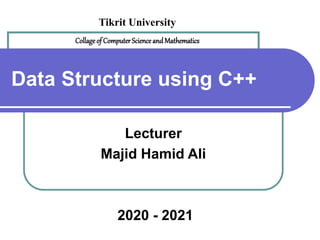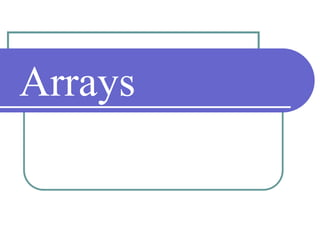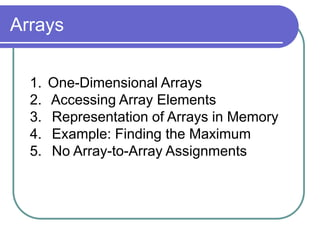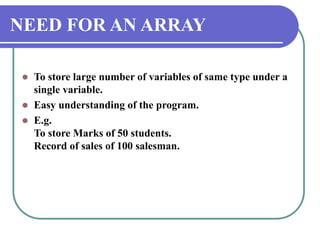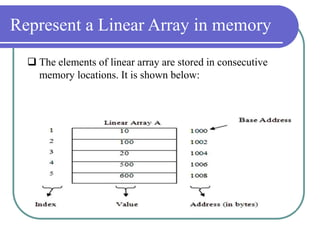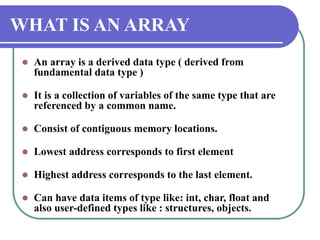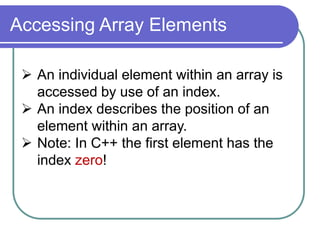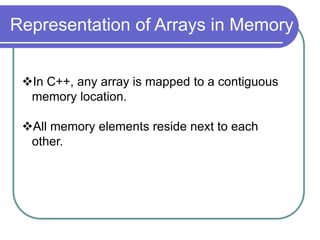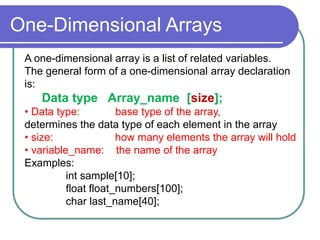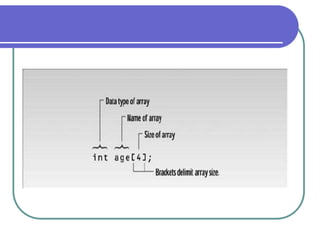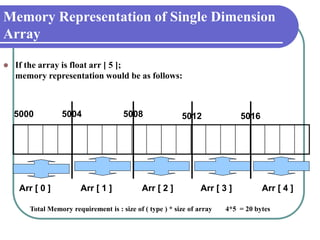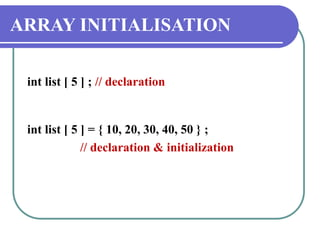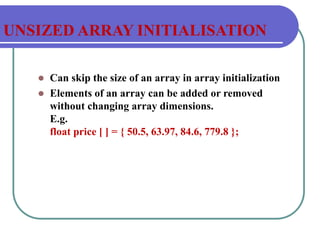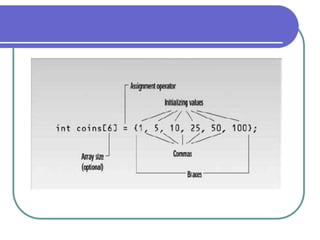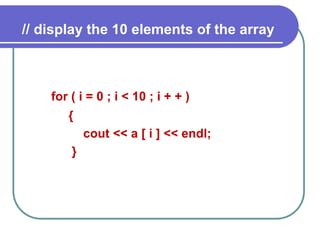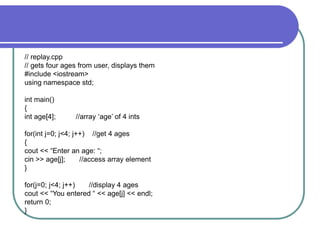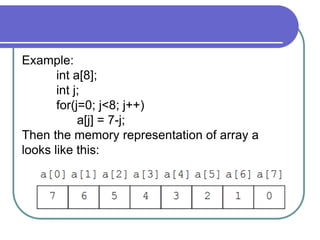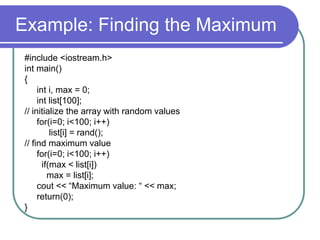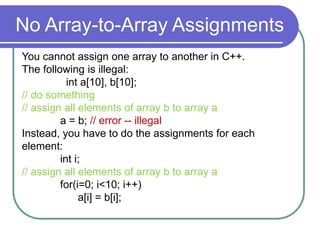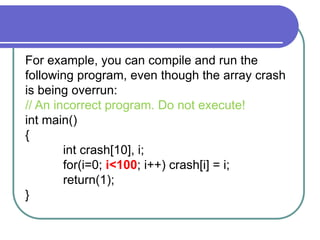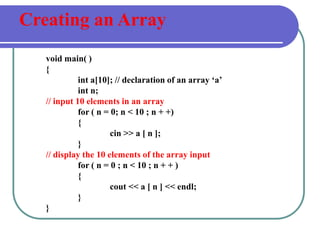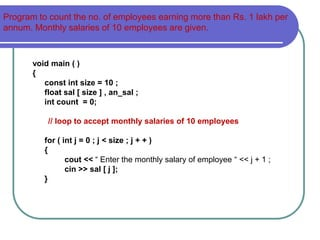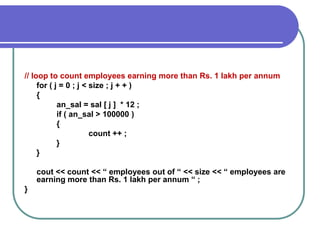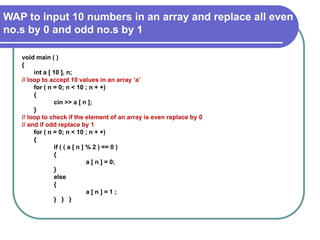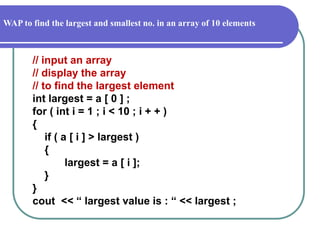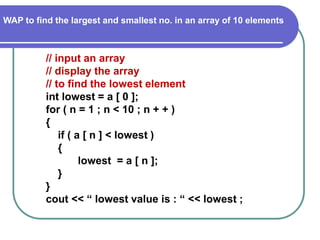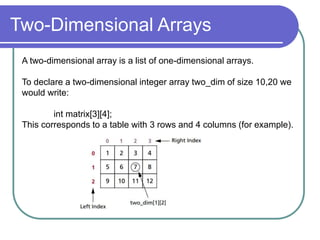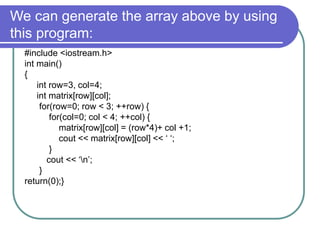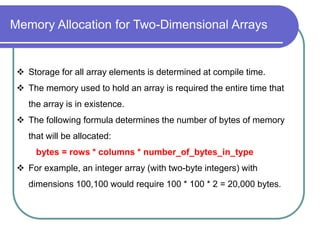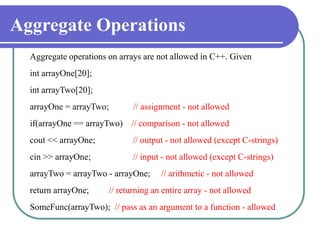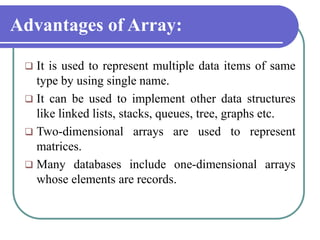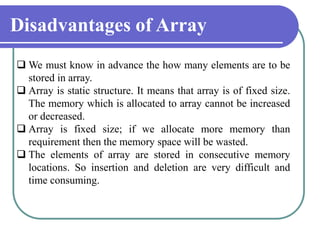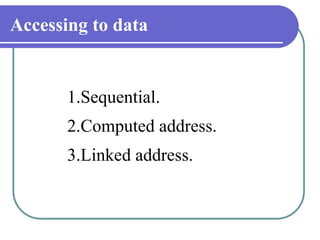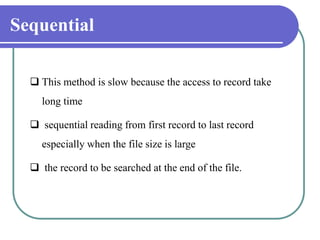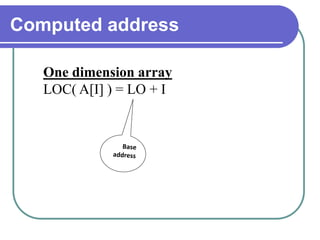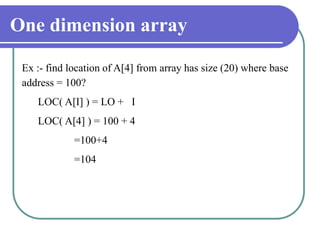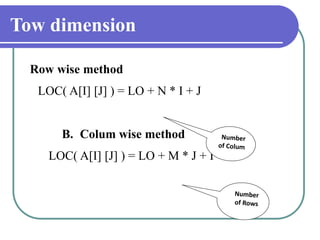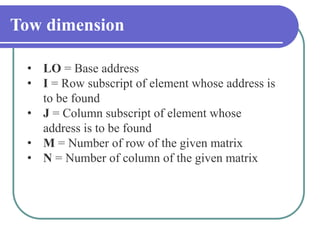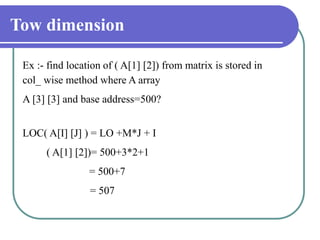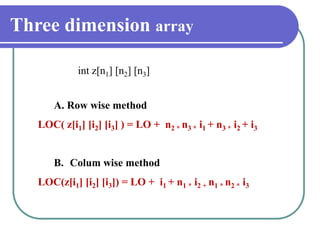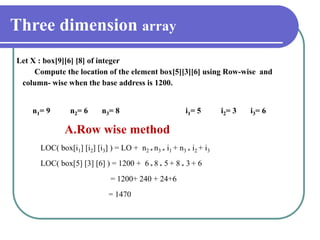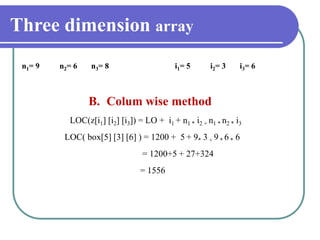1 sur 42
Publicité

### Data structure array

1. Data Structure using C++ Lecturer Majid Hamid Ali 2020 - 2021 Tikrit University Collageof ComputerScienceandMathematics
2. Arrays
3. Arrays 1. One-Dimensional Arrays 2. Accessing Array Elements 3. Representation of Arrays in Memory 4. Example: Finding the Maximum 5. No Array-to-Array Assignments
4. NEED FOR AN ARRAY  To store large number of variables of same type under a single variable.  Easy understanding of the program.  E.g. To store Marks of 50 students. Record of sales of 100 salesman.
5. Represent a Linear Array in memory  The elements of linear array are stored in consecutive memory locations. It is shown below:
6. WHAT IS AN ARRAY  An array is a derived data type ( derived from fundamental data type )  It is a collection of variables of the same type that are referenced by a common name.  Consist of contiguous memory locations.  Lowest address corresponds to first element  Highest address corresponds to the last element.  Can have data items of type like: int, char, float and also user-defined types like : structures, objects.
7. Accessing Array Elements  An individual element within an array is accessed by use of an index.  An index describes the position of an element within an array.  Note: In C++ the first element has the index zero!
8. Representation of Arrays in Memory In C++, any array is mapped to a contiguous memory location. All memory elements reside next to each other.
9. One-Dimensional Arrays A one-dimensional array is a list of related variables. The general form of a one-dimensional array declaration is: Data type Array_name [size]; • Data type: base type of the array, determines the data type of each element in the array • size: how many elements the array will hold • variable_name: the name of the array Examples: int sample; float float_numbers; char last_name;
10. Memory Representation of Single Dimension Array  If the array is float arr [ 5 ]; memory representation would be as follows: Arr [ 0 ] Arr [ 1 ] Arr [ 2 ] Arr [ 3 ] Arr [ 4 ] 5016 5012 5008 5004 5000 Total Memory requirement is : size of ( type ) * size of array 4*5 = 20 bytes
11. ARRAY INITIALISATION int list [ 5 ] ; // declaration int list [ 5 ] = { 10, 20, 30, 40, 50 } ; // declaration & initialization
12. UNSIZED ARRAY INITIALISATION  Can skip the size of an array in array initialization  Elements of an array can be added or removed without changing array dimensions. E.g. float price [ ] = { 50.5, 63.97, 84.6, 779.8 };
13. for ( i = 0 ; i < 10 ; i + + ) { cout << a [ i ] << endl; } // display the 10 elements of the array
14. // replay.cpp // gets four ages from user, displays them #include <iostream> using namespace std; int main() { int age; //array ‘age’ of 4 ints for(int j=0; j<4; j++) //get 4 ages { cout << “Enter an age: “; cin >> age[j]; //access array element } for(j=0; j<4; j++) //display 4 ages cout << “You entered “ << age[j] << endl; return 0; }
15. Example: int a; int j; for(j=0; j<8; j++) a[j] = 7-j; Then the memory representation of array a looks like this:
16. Example: Finding the Maximum #include <iostream.h> int main() { int i, max = 0; int list; // initialize the array with random values for(i=0; i<100; i++) list[i] = rand(); // find maximum value for(i=0; i<100; i++) if(max < list[i]) max = list[i]; cout << “Maximum value: “ << max; return(0); }
17. No Array-to-Array Assignments You cannot assign one array to another in C++. The following is illegal: int a, b; // do something // assign all elements of array b to array a a = b; // error -- illegal Instead, you have to do the assignments for each element: int i; // assign all elements of array b to array a for(i=0; i<10; i++) a[i] = b[i];
18. For example, you can compile and run the following program, even though the array crash is being overrun: // An incorrect program. Do not execute! int main() { int crash, i; for(i=0; i<100; i++) crash[i] = i; return(1); }
19. Creating an Array void main( ) { int a; // declaration of an array ‘a’ int n; // input 10 elements in an array for ( n = 0; n < 10 ; n + +) { cin >> a [ n ]; } // display the 10 elements of the array input for ( n = 0 ; n < 10 ; n + + ) { cout << a [ n ] << endl; } }
20. Program to count the no. of employees earning more than Rs. 1 lakh per annum. Monthly salaries of 10 employees are given. void main ( ) { const int size = 10 ; float sal [ size ] , an_sal ; int count = 0; // loop to accept monthly salaries of 10 employees for ( int j = 0 ; j < size ; j + + ) { cout << “ Enter the monthly salary of employee “ << j + 1 ; cin >> sal [ j ]; }
21. // loop to count employees earning more than Rs. 1 lakh per annum for ( j = 0 ; j < size ; j + + ) { an_sal = sal [ j ] * 12 ; if ( an_sal > 100000 ) { count ++ ; } } cout << count << “ employees out of “ << size << “ employees are earning more than Rs. 1 lakh per annum “ ; }
22. WAP to input 10 numbers in an array and replace all even no.s by 0 and odd no.s by 1 void main ( ) { int a [ 10 ], n; // loop to accept 10 values in an array ‘a’ for ( n = 0; n < 10 ; n + +) { cin >> a [ n ]; } // loop to check if the element of an array is even replace by 0 // and if odd replace by 1 for ( n = 0; n < 10 ; n + +) { if ( ( a [ n ] % 2 ) == 0 ) { a [ n ] = 0; } else { a [ n ] = 1 ; } } }
23. WAP to find the largest and smallest no. in an array of 10 elements // input an array // display the array // to find the largest element int largest = a [ 0 ] ; for ( int i = 1 ; i < 10 ; i + + ) { if ( a [ i ] > largest ) { largest = a [ i ]; } } cout << “ largest value is : “ << largest ;
24. WAP to find the largest and smallest no. in an array of 10 elements // input an array // display the array // to find the lowest element int lowest = a [ 0 ]; for ( n = 1 ; n < 10 ; n + + ) { if ( a [ n ] < lowest ) { lowest = a [ n ]; } } cout << “ lowest value is : “ << lowest ;
25. Two-Dimensional Arrays A two-dimensional array is a list of one-dimensional arrays. To declare a two-dimensional integer array two_dim of size 10,20 we would write: int matrix; This corresponds to a table with 3 rows and 4 columns (for example).
26. We can generate the array above by using this program: #include <iostream.h> int main() { int row=3, col=4; int matrix[row][col]; for(row=0; row < 3; ++row) { for(col=0; col < 4; ++col) { matrix[row][col] = (row*4)+ col +1; cout << matrix[row][col] << ‘ ‘; } cout << ‘n’; } return(0);}
27. Memory Allocation for Two-Dimensional Arrays  Storage for all array elements is determined at compile time.  The memory used to hold an array is required the entire time that the array is in existence.  The following formula determines the number of bytes of memory that will be allocated: bytes = rows * columns * number_of_bytes_in_type  For example, an integer array (with two-byte integers) with dimensions 100,100 would require 100 * 100 * 2 = 20,000 bytes.
28. Aggregate Operations Aggregate operations on arrays are not allowed in C++. Given int arrayOne; int arrayTwo; arrayOne = arrayTwo; // assignment - not allowed if(arrayOne == arrayTwo) // comparison - not allowed cout << arrayOne; // output - not allowed (except C-strings) cin >> arrayOne; // input - not allowed (except C-strings) arrayTwo = arrayTwo - arrayOne; // arithmetic - not allowed return arrayOne; // returning an entire array - not allowed SomeFunc(arrayTwo); // pass as an argument to a function - allowed
29. Advantages of Array:  It is used to represent multiple data items of same type by using single name.  It can be used to implement other data structures like linked lists, stacks, queues, tree, graphs etc.  Two-dimensional arrays are used to represent matrices.  Many databases include one-dimensional arrays whose elements are records.
30. Disadvantages of Array  We must know in advance the how many elements are to be stored in array.  Array is static structure. It means that array is of fixed size. The memory which is allocated to array cannot be increased or decreased.  Array is fixed size; if we allocate more memory than requirement then the memory space will be wasted.  The elements of array are stored in consecutive memory locations. So insertion and deletion are very difficult and time consuming.